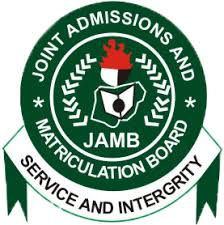HomeJambWaecNecoNabtebNews
Welccome To Examloaded.Com>>>>  Nigeria's No 1 Exam Expo Website, With 7 Years + of Experience Verified by Google #Na We Sure Pass!
Our Only Contact | Call | Text| WhatsApp08162563540

SUBSCRIBE HERE NOW!!# WAEC GCE/WASSCE NOV/DEC 2018/2019 LIKELY QUESTIONS AND ANSWERS FOR GENERAL MATHEMATICS

The West African Examinations Council (WAEC) is an examination board that conducts the West African Senior School Certificate Examination, for University and Jamb entry examination in West Africa countries. … In a year, over three million candidates registered for the exams coordinated by WAEC.

WAEC GCE Mathematics Questions

Question 1

A = {2, 4, 6, 8}, B = {2, 3, 7, 9} and C = {x: 3 < x h = .J(10.5)2 – (3.5)2 = 9.90cm

22 x 10.5

Volume of cone = .1 x 22 x 3.5 x 9.899 == 127 cm²

3 7 1.

Question 5.

Two fair dice are thrown.

M is the event described by “the sum of the scores is 10” and

N is the event described by “the difference between the scores is 3”.

(a) Write out the elements of M and N.

(b) Find the probability of M or N.

(c) Are M and N mutually exclusive? Give reasons.

Observation.Always remember to list the elements of M and N as required.

Furthermore make sure you state correctly the condition for which events are mutually exclusive.

You are also to show that M = { (4,6) , (5,5), (6,4}. N = { (1,4), (2,5), (3,6), (4,1),

(5,2), (6,3) }. Probability of M = 3/36 = 1/12. Probability of N = 6/36 = 1/6.

Probability of Nor M:1/12 + 1/6 = 1/4. M and N are mutually exclusive because noelement is common to both sets. Thus the two events cannot happen at the same time i.e. n(M n N) = O.
Question 6.

(a) The scale of a map is 1:20,000. Calculate the area, in square centimetres, on the map of a forest reserve which covers 85 km²,

(b) A rectangular playing field is 18 m wide. It is surrounded by a path 6m wide such that its area is equal to the area of the path. Calculate the length of the field.

(c)

lkm = 100,000 ern, therefore 1km2 = 1km x 1km = 100,000 x 100,000 = 10,000 000,OOOcm2•

85km2 = 850,000,000,000 cm². 20,000 em on the ground = 1 cm on the map, hence 400,000,000 cm² on the ground = 1 cm2850,000,000,000 = 2125 cm2 on the map. 400,000,000.

Candidates should draw the diagram correctly.

From the diagram, area of path = 2(30 x 6) + 12a = 360 + 12a. Area of field = 18a. Since they are equal, 18a = 12a + 360. This gave a = 60 m.

see the reflex angle as 360 – x, hence, did not subtract their final answer from 360° when they had calculated the value of the reflex angle. Here, 360 – x x 22x Z x Z = 27.5 cm2

360 7 2 2

This meant that 360 – x = 360 x 2 x 27.5 from where we obtain x = 103° to the nearest degree

77.

Question 8

Using ruler and a pair of compasses only,

(a) construct

(i) a quadrilateral PQRS with IPSI :: 6 cm, LRSP:: 90°,

IRSI = 9 ern, IQRI = 8.4 cm and IPQI :: 5.4 cm;

(ii) the bisectors of LRSP and LSPQ to meet at X;

(iii) The perpendicular XTto meet PS at T.

(b) Measure IXT/’

Math waec gce 2017 Observation.Please construct the angle and not measure them.

construct a perpendicular from a given point to a given line segment.

Question 9.

In the diagram, /AB/ = 8 km, /BC/ = 13 km, the bearing of A from B is 310° and the bearing of B from C is 230°. Calculate, correct to 3 significant figures,

(a) the distance AC;

(b) the bearing of C from A;

(c) how far east of B, C is